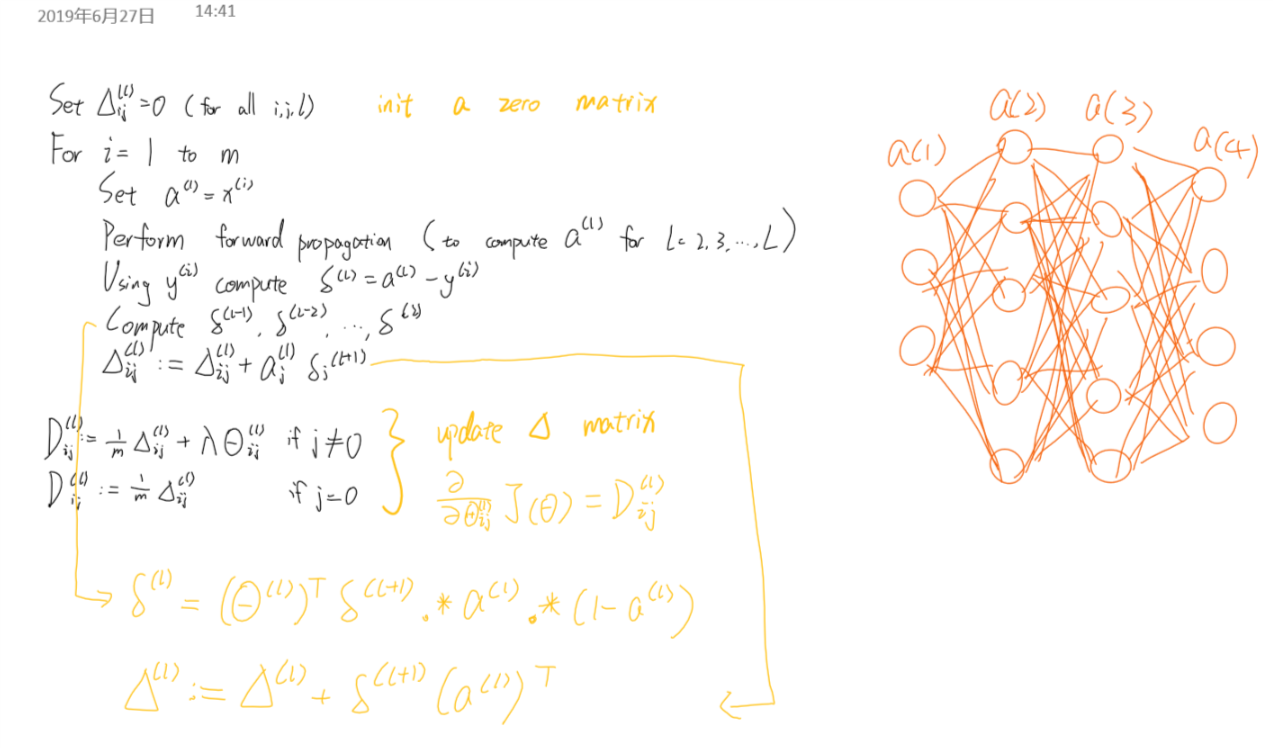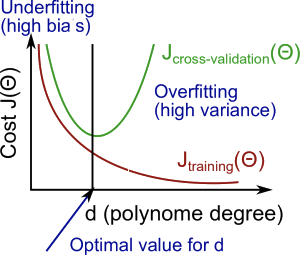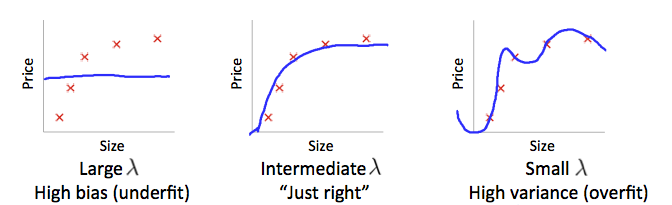# ML Course Notes 03

## Lecture 09: Classification and Representation

### Cost Function

Recall that the cost function for regularized logistic regression was: $J(\theta)=-\frac{1}{m} \sum_{i=1}^{m}\left[y^{(i)} \log \left(h \theta\left(x^{(i)}\right)\right)+\left(1-y^{(i)}\right) \log \left(1-h \theta\left(x^{(i)}\right)\right)\right]+\frac{\lambda}{2 m} \sum_{j=1}^{n} \theta_{j}^{2}$

For neural networks, it is going to be: $J(\Theta)=-\frac{1}{m} \sum_{i=1}^{m} \sum_{k=1}^{K}\left[y_{k}^{(i)} \log \left(\left(h_{\Theta}\left(x^{(i)}\right)\right)_{k}\right)+\left(1-y_{k}^{(i)}\right) \log \left(1-\left(h_{\Theta}\left(x^{(i)}\right)\right)_{k}\right)\right]+\frac{\lambda}{2 m} \sum_{l=1}^{L-1} \sum_{j=1}^{s l} \sum_{j=1}^{s l_{l} 1}\left(\Theta_{j, i}^{(l)}\right)^{2}$

• $L$ = total number of layers in the network
• $S_l$ = number of units (not counting bias unit) in layer l
• $K$ = number of output units/classes
• the double sum simply adds up the logistic regression costs calculated for each cell in the output layer
• the triple sum simply adds up the squares of all the individual Θs in the entire network.
• the i in the triple sum does not refer to training example i

### Backpropagation Algorithm

• Aim : $min_\Theta J(\Theta)$
• need to compute $\dfrac{\partial}{\partial \Theta_{i,j}^{(l)}}J(\Theta)$
• Algorithm### Backpropagation In Practice

pick a network architecture: choose the layout of your neural network, including how many hidden units in each layer and how many layers in total you want to have.

• Number of input units = dimension of features $x^{(i)}$
• Number of output units = number of classes
• Number of hidden units per layer = usually more the better (must balance with cost of computation as it increases with more hidden units)
• Defaults: 1 hidden layer. If you have more than 1 hidden layer, then it is recommended that you have the same number of units in every hidden layer.

Training a Neural Network

1. Randomly initialize the weights
2. Implement forward propagation to get $h_Θ(x(i))$ for any $x^{(i)}$
3. Implement the cost function
4. Implement backpropagation to compute partial derivatives
6. Use gradient descent or a built-in optimization function to minimize the cost function with the weights in theta.

pass;

## Lecture10: Advice for applying machine learning

### What to do next?

Once we have done some trouble shooting for errors in our predictions by:

• Get more training examples
• Try smaller sets of features
• Try decreasing $\lambda$
• Try increasing $\lambda$

### Evaluating a hypothesis

Calculate $J_{test}$ (Maybe named it $J_{validate}$ is better) and $J_{train}$ to judge if it’s overfitting or underfitting.

However, with my own experience and understanding, the overfitting and underfitting appears at every models, no matter if it’s a nice one. You only have to judge if it’s too much. Overfitting and underfitting actually shows the unity of opposites. Basic ML models’ working is in such a situation in which the predict and fit depends on the co-existence of overfitting and underfitting which are opposite to each other, remain dependent on each other and presupposing each other within a field of tension.

### Model Selection and Train/Validation/Test Sets

We can now calculate three separate error values for the three different sets using the following method:

1. Optimize the parameters in Θ using the training set for each polynomial degree.
2. Find the polynomial degree d with the least error using the cross validation set.
3. Estimate the generalization error using the test set with $J_{test}(\Theta^{(d)})$, ($d$ = theta from polynomial with lower error);

### Diagnosing Bias vs. Variance

The training error will tend to decrease as we increase the degree d of the polynomial.

At the same time, the cross validation error will tend to decrease as we increase d up to a point, and then it will increase as d is increased, forming a convex curve.

• High bias (underfitting): both $J_{train}(\Theta)$ and $J_{CV}(\Theta)$ will be high. Also, $J_{train}(\Theta)\approx J_{CV}(\Theta)$.
• High variance (overfitting): $J_{train}(\Theta)$ will be low and $J_{CV}(\Theta)$ will be much greater than $J_{train}(\Theta)$.### Regularization and Bias/VarianceAs the situationshows above, in order to choose the model and the regularization term $\lambda$, we need to:

1. Create a list of lambdas (i.e. $\lambda$∈{0,0.01,0.02,0.04,0.08,0.16,0.32,0.64,1.28,2.56,5.12,10.24});
2. Create a set of models with different degrees or any other variants.
3. Iterate through the $\lambda_s$ and for each $\lambda_{go}$ through all the models to learn some Θ.
4. Compute the cross validation error using the learned Θ (computed with $\lambda$) on the $J_{CV}(Θ)$ without regularization or $\lambda$ = 0.
5. Select the best combo that produces the lowest error on the cross validation set.
6. Using the best combo $\Theta$ and $\lambda$, apply it on $J_{test}(\Theta)$ to see if it has a good generalization of the problem.

### Learning Curves

Professor Ng talks about lots of situations and anyalyze how training set size effect the performance in overfitting and underfitting situation during this part. (It’s difficult to do rote memorization but you will easily figuare out how the performance change in the above situations if you have some experience about ML, even very few experience also works.)

• If a learning algorithm is suffering from high bias, getting more training data will not (by itself) help much.
• If a learning algorithm is suffering from high variance, getting more training data is likely to help.

#### Deciding What to Do Next Revisited

Our decision process can be broken down as follows:

• Getting more training examples: Fixes high variance
• Trying smaller sets of features: Fixes high variance
• Adding features: Fixes high bias
• Adding polynomial features: Fixes high bias
• Decreasing λ: Fixes high bias
• Increasing λ: Fixes high variance.

Diagnosing Neural Networks

• A neural network with fewer parameters is prone to underfitting. It is also computationally cheaper.
• A large neural network with more parameters is prone to overfitting. It is also computationally expensive. In this case you can use regularization (increase λ) to address the overfitting.

Using a single hidden layer is a good starting default. You can train your neural network on a number of hidden layers using your cross validation set. You can then select the one that performs best.

Model Complexity Effects:

• Lower-order polynomials (low model complexity) have high bias and low variance. In this case, the model fits poorly consistently.
• Higher-order polynomials (high model complexity) fit the training data extremely well and the test data extremely poorly. These have low bias on the training data, but very high variance.
• In reality, we would want to choose a model somewhere in between, that can generalize well but also fits the data reasonably well.

## Lecture11: Machine learning system design

Professor Ng talks about some specific examplesduring this part, so I just pass noting most of them.

The recommended approach to solving machine learning problems is to:

• Start with a simple algorithm, implement it quickly, and test it early on your cross validation data.
• Plot learning curves to decide if more data, more features, etc. are likely to help.
• Manually examine the errors on examples in the cross validation set and try to spot a trend where most of the errors were made.

It is very important to get error results as a single, numerical value.

Hence, we should try new things, get a numerical value for our error rate, and based on our result decide whether we want to keep the new feature or not.